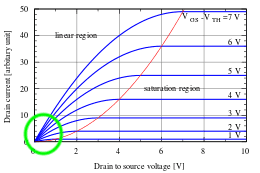# MOSFET Transistors as Switches

• Fascheue
In summary, the conversation discusses the behavior of MOSFETs in the triode region and the equations that describe their behavior. The first image shows that the drain current will increase as the gate-source voltage increases, while the second image implies that the drain current remains constant. This discrepancy is due to the different models being used in the two images - in the triode region, the MOSFET is modeled as a resistor and the current depends on the gate-source voltage, while in the saturation region, the current is limited by the resistance of the bulk material and the applied voltage. The second image also suggests that the resistor model holds when the gate voltage is sufficiently high. However, the value of the resistance will decrease as the gate-source voltagef

#### Fascheue

I’ve attached two images from my textbook. One describing how MOSFEET’s act like small resistances when in the triode region and open circuits when in the cutoff region, the other a list of equations describing the behavior of an NMOS transistor. I’m having trouble making sense of these two pages.

In the triode region, does the drain current depend on the gate-source voltage? These to pages seem to suggest different answers to that question. In the first image, it looks like drain current will increase as the gate-source voltage increases. In the second image, it looks like drain current remains constant as the gate-source voltage increases. drain current seemingly only depends of the drain-source voltage in this case.

Any clarification would be helpful, thanks.

#### Attachments

In both the triode region and in the saturation region, the drain current will increase as the gate-source voltage increases. What in the second image made you think otherwise?

•Fascheue
In both the triode region and in the saturation region, the drain current will increase as the gate-source voltage increases. What in the second image made you think otherwise?
The MOSFET in the triode region is being modeled as a resistor. From ohm’s law, the current across that resistor in the second image should be equal to V/R, where V is the drain-source voltage. In the equation I = Vds/R, there is no gate-source voltage dependency.

And isn’t the second image suggesting that this resistor model holds whenever the gate voltage is sufficiently high?

The MOSFET in the triode region is being modeled as a resistor. From ohm’s law, the current across that resistor in the second image should be equal to V/R, where V is the drain-source voltage. In the equation I = Vds/R, there is no gate-source voltage dependency.

And isn’t the second image suggesting that this resistor model holds whenever the gate voltage is sufficiently high?
The value of that resistance will drop as the gate-source voltage is increased, at least until it becomes limited by the resistance of the wires, etc.

•Fascheue
If you look at the I-V characteristics down near rhe origin, it can basically be considered as a voltage controlled resistor, where the resistance is a function of the gate-source voltage.•Fascheue
In the first image, it looks like drain current will increase as the gate-source voltage increases. In the second image, it looks like drain current remains constant as the gate-source voltage increases. drain current seemingly only depends of the drain-source voltage in this case.
Simple answer: They are both correct, but under different conditions.

The details:
In the first case, the device in operating in the linear, or triode, region. The Gate-Source voltage (VGS) is such that it is partially pinching off the Conduction Channel between Source and Drain. As the Conduction Channel varies in size with the VGS changes, the effective resistance changes... just as a smaller wire has a higher resistance than a large diameter wire. (With a little hand-waving), this is how you get amplification, by having a voltage on the gate controlling how much current flows between Source and Drain.

In the second case, the VGS is such that the conduction channel is at its maximum size, and the current is limited by the resistance of the bulk Source-Drain material and the applied Source-Drain voltage. Since this is a fixed resistance, the current is determined by the applied voltage.

Hopes this helps!

Cheers,
Tom

•essenmein